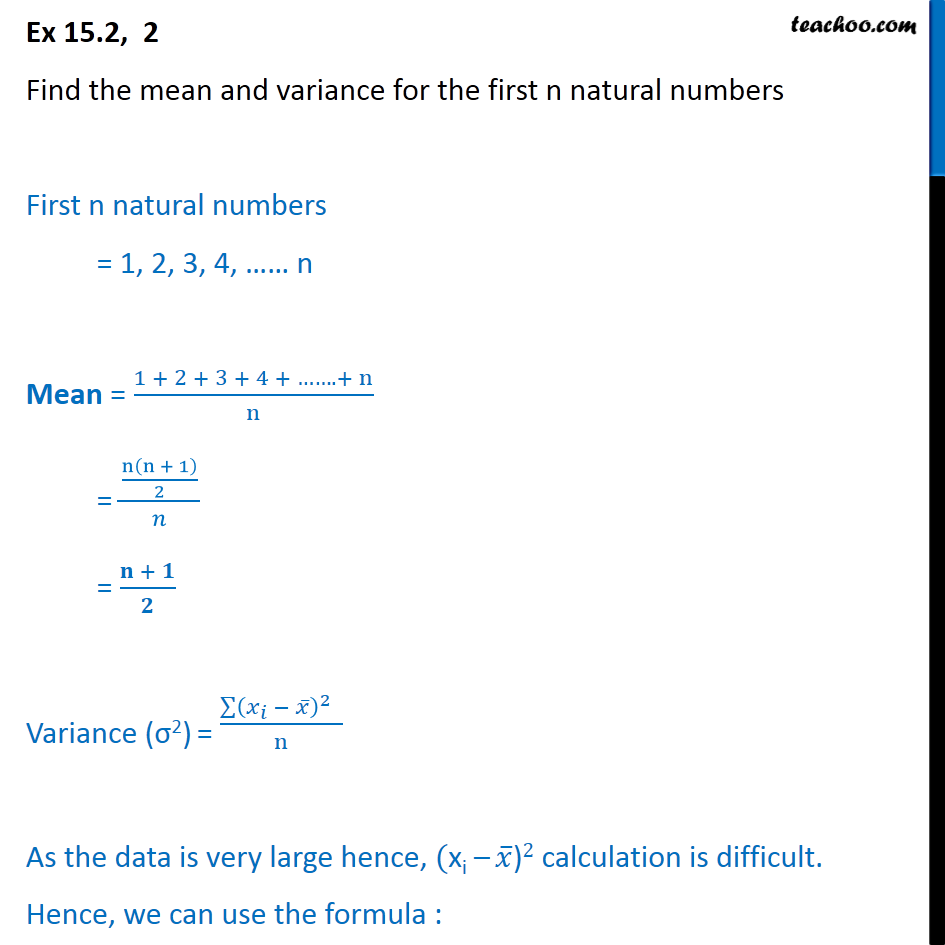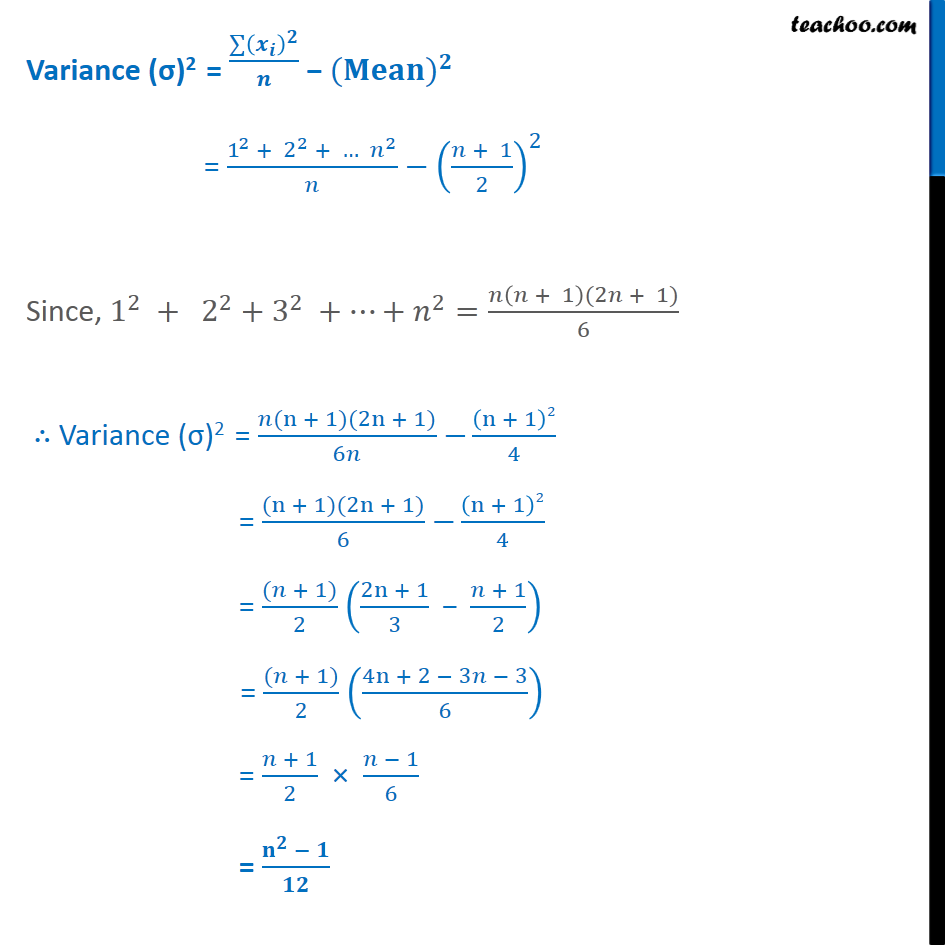Ex 13.2

Chapter 13 Class 11 Statistics
Serial order wiseLearn in your speed, with individual attention - Teachoo Maths 1-on-1 Class

### Transcript

Ex 13.2, 2 Find the mean and variance for the first n natural numbers First n natural numbers = 1, 2, 3, 4, …… n Mean = (1 + 2 + 3 + 4 + ……. + n)/n = (n(n + 1)/2)/𝑛 = (𝐧 + 𝟏)/𝟐 Variance (σ2) = (∑▒(𝑥_𝑖 − 𝑥 ̅ )^2 )/n As the data is very large hence, "(xi – " 𝑥 ̅")" 2 calculation is difficult. Hence, we can use the formula : ∴ Variance (σ)2 = (𝑛(n + 1)(2n + 1))/6𝑛− (n + 1)2/4 = ((n + 1)(2n + 1))/6− (n + 1)2/4 = ((𝑛 + 1))/2 ((2n + 1)/3 " − " (𝑛 + 1)/2) = ((𝑛 + 1))/2 ((4n + 2 − 3𝑛 − 3)/6) = (𝑛 + 1)/2 × (𝑛 − 1)/6 = (𝐧^𝟐 − 𝟏)/𝟏𝟐